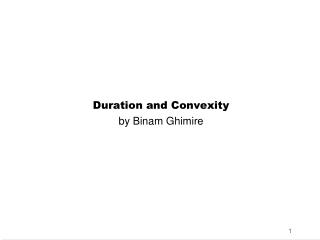DownloadDownload PresentationDuration and Convexity by Binam Ghimire

# Duration and Convexity by Binam Ghimire

Download Presentation## Duration and Convexity by Binam Ghimire

- - - - - - - - - - - - - - - - - - - - - - - - - - - E N D - - - - - - - - - - - - - - - - - - - - - - - - - - -
##### Presentation Transcript

1. Duration and Convexity by BinamGhimire

2. Learning Objectives • Duration of a bond, how to compute it • Modified duration and the relationship between a bond’s modified duration and its volatility • Convexity for a bond, and computation • Under what conditions is it necessary to consider both modified duration and convexity when estimating a bond’s price volatility? • Excel computation

3. Duration • Developed by Frederick Macaulay, 1938 • It combines the properties of maturity and coupon

4. Duration • Example • Two 20 – year bonds, one with an 8% coupon and the other with a 15% coupon, do not have identical life economic times. An investor will recover the original purchase price much sooner with the 15% coupon bond. • Therefore a measure is needed that accounts for the entire pattern (both size and timing) of the cashflows over the life of the bond – the effective maturity of the bond. Such a concept is called Duration

5. Duration Where: t = time period in which the coupon or principal payment occurs Ct= interest or principal payment that occurs in period t i = yield to maturity on the bond

6. Duration • Duration is the average number of years an investor waits to get the money back. • Duration is the weighted average, on a present value basis, of the time to full recovery of the principal and interest payment on a bond.

7. Duration • Calculation of Duration depends on 3 factors • The Coupon Payments • Time to Maturity • The YTM

8. Duration • The Coupon of Payments • Coupon is ………….related to duration. This is logical because higher coupons lead to …………….. recovery of the bond’s value resulting in a ………… duration, relative to lower coupons

9. Duration • The Coupon of Payments • Coupon is inversely related to duration. This is logical because higher coupons lead to quicker recovery of the bond’s value resulting in a shorter duration, relative to lower coupons

10. Duration • Time to Maturity • Duration ………………. with time to maturity but a decreasing rate

11. Duration • Time to Maturity • Duration expands with time to maturity but a decreasing rate

12. Duration • Time to Maturity • Note that for all coupon paying bonds, duration is always less than maturity. • For a zero coupon bond, duration is equal to maturity

13. Duration • YTM • YTM is inversely related to duration

14. Characteristics of Duration • Duration of a bond with coupons is always less than its term to maturity because duration gives weight to these interim payments • A zero-coupon bond’s duration equals its maturity • There is an inverse relation between duration and coupon

15. Characteristics of Duration • There is a positive relation between term to maturity and duration, but duration increases at a decreasing rate with maturity • There is an inverse relation between YTM and duration • Sinking funds and call provisions can have a dramatic effect on a bond’s duration

16. Modified Duration and Bond Price Volatility An adjusted measure of duration can be used to approximate the price volatility of a bond Where: m = number of payments a year YTM = nominal YTM

17. Duration and Bond Price Volatility • Bond price movements will vary proportionally with modified duration for small changes in yields • An estimate of the percentage change in bond prices equals the change in yield time modified duration Where: P = change in price for the bond P = beginning price for the bond Dmod = the modified duration of the bond i = yield change in basis points divided by 100

18. Convexity • The equation above generally provides an approximate change in price for very small changes in required yield. However, as changes become larger, the approximation becomes poorer. • Modified duration merely produces symmetric percentage price change estimates using equation when, in actuality, the price-yield relationship is not linear but curvilinear. (pls see price-yield graph already covered) • Hence, Convexity is the term used to refer to the degree to which duration changes as the YTM changes.

19. Convexity • Convexity is largest for low coupon bonds, long-maturity bonds, and low YTM.

20. Convexity The convexity is the measure of the curvature and is the second derivative of price with resect to yield (d2P/di2) divided by price Convexity is the percentage change in dP/di for a given change in yield

21. Convexity • Inverse relationship between coupon and convexity • Direct relationship between maturity and convexity • Inverse relationship between yield and convexity